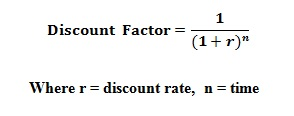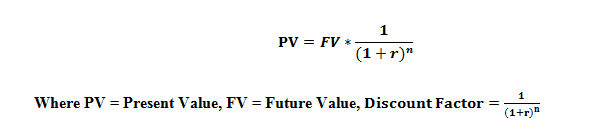# How to Calculate Discount Rate

## What is Discount Rate

Discount factor or the discount rate can be identified as an essential component for the organizations for important decision making in projects. Many businesses are using the discount factor to calculate the present value of future cash flows of the proposed projects in order to do a cost benefit analysis. Before undertaking the projects, the companies are used to check whether they are financially feasible or not.

The discount rate can be expressed as the interest rate that is applied in calculating the present value of the future cash flows. In accounting, a mathematical formula has been used to calculate the discount factor. The discount factor is made up of two elements such as time and the discount rate.

## Discount Factor Formula

The discount factor formula can be illustrated as follows:In order to calculate discount rate, there are various methods that have been used such as the weighted average cost of capital (WACC) and the capital asset pricing model (CAPM), hurdle rate method and Gordon model. Time duration (n) of the discount factor expresses the expected period of the future cash flows.

For financial accounting purposes, the discount factor has been useful in many ways. In preparing the financial statements, future cash flows cannot be included mainly because of the revenue recognition principle and the cost principle. According to those principles, all the transactions must be recorded with their exact cash values at the time of which the transaction takes place. In order to adhere to those principles, the discount factor has been used by the accountants to find the present value of the future cash flows and that value has been recorded in the financial statements at the time of the transaction.

The present value of the future cash flows can be calculated using the below formula:Not only for the accounting purposes, the discount factor has been beneficial for economic analysis too. It has been useful to get a clear idea about the proposed projects. It also can be used to identify the areas that need to be highly concentrated on.

When concerning about the limitations associated with the discount factor calculations, time and discount rates are uncertain. Discount rate may fluctuate depending on various economical factors. Therefore, these changes may have an impact on the calculated financial figures. Further, the time is also an unpredictable factor as unexpected incidents may have a negative impact on the projects future cash flows.